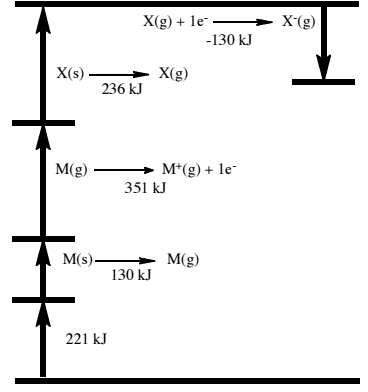# Problem: Please refer to the hypothetical Born-Haber cycle below for M(s) + X(s) → MX(s), where M and X are both elements in their standard states.  The enthalpy of formation (ΔH f) for MX(s) is: a. 938 kJ     b. 130 kJ     c. 808 kJ     d. 221 kJ     e. -221 kJ

###### FREE Expert Solution
98% (208 ratings)
###### Problem Details

Please refer to the hypothetical Born-Haber cycle below for M(s) + X(s) → MX(s), where M and X are both elements in their standard states.

The enthalpy of formation (ΔH f) for MX(s) is:

a. 938 kJ     b. 130 kJ     c. 808 kJ     d. 221 kJ     e. -221 kJ